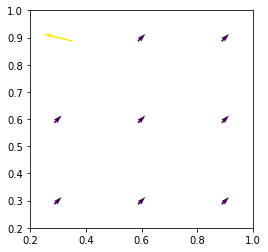# Quiver not drawing as expected

Hi, perhaps I am misinterpreting the docs. When I run the following code, I expect the bottom-right arrow (element [2,0]) to point to the left. However, it’s the top-left as (see figure). Quiver seems to be transposing the U,V matrices. Am I missing anything?

``````import numpy as np
import matplotlib.pyplot as plt

x = np.linspace(0.3,.9,3)
y = x

nb_points = x.size
X,Y = np.meshgrid(x,y)

U = np.ones((nb_points, nb_points))
V = np.ones((nb_points, nb_points))
U[2,0] = -3
M = (np.hypot(U, V))

fig,ax = plt.subplots(1,1, figsize=(4,4))
ax.quiver(X, Y, U, V, M, units='width', pivot='middle')
ax.set_xlim(.2,1)
ax.set_ylim(.2,1)
``````Got it. I completely misinterpreted the meaning of U,V’s indeces

U[2,0] is supposed to be interpreted as the direction of X[2,0],Y[2,0] = (.3,.9), which is the top-left point

2 Likes